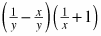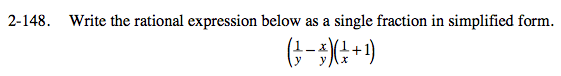### Home > PC > Chapter 2 > Lesson 2.3.6 > Problem2-148

2-148.

Write the rational expression below as a single fraction in simplified form. Homework Help ✎Rewrite each factor with a common denominator.

Multiply the fractions.

Simplify.

$\left(\frac{1-x}{y}\right)\left(\frac{1+x}{x}\right)$

$\frac{1-x+x-x^2}{xy}$

$\frac{1-x^2}{xy}$